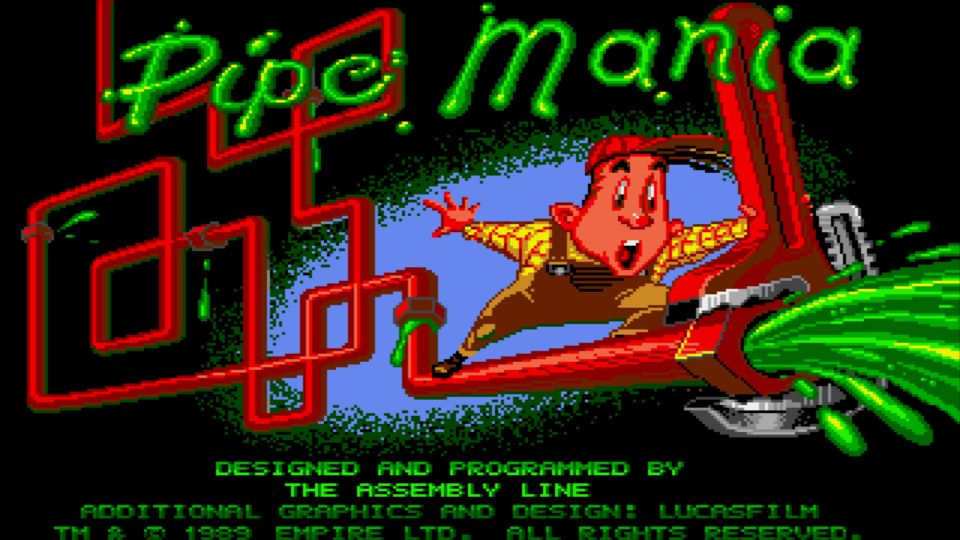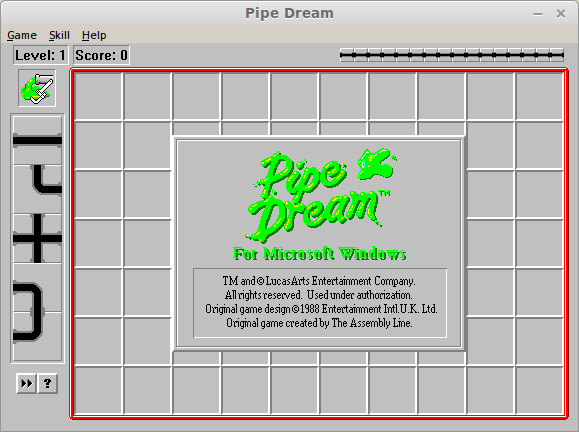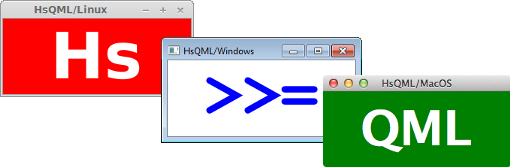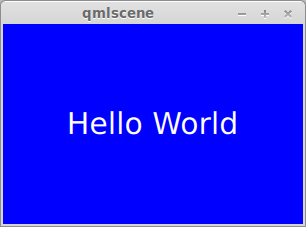# Data Modelling# Modelling with Types

``````
data Bearing
``````    = North
``````    | East
``````    | South
``````    | West
``````

## Tile Data Type

``````
data Tile
``````    = Start Bearing
``````

``````

## Other Tile Constructors``````
data Tile
= Start Bearing
``````    | End Bearing
``````    | Corner Bearing
``````    | Straight Orientation
``````    | Cross
``````
``````
data Orientation
= Horizontal
| Vertical
``````

## `Map Point Tile`

``````
Map.fromList [(Point 1 3, Start North),
(Point 1 2, Straight Vertical),
(Point 1 1, Corner East),
(Point 2 1, Cross),
(Point 4 4, Corner North)]
``````

### The Point data type is simply defined as:

``````
data Point = Point Int Int
``````# Key Observeration

## Sewage enters and exits each tile

``````
data Plumb = Plumb Bearing Bearing
``````

## The Start and End tiles

``````
data Plumb = Plumb (Maybe Bearing) (Maybe Bearing)
``````

### Maybe is a polymorphic data type representing the presence or absence of a value

``````
data Maybe a = Just a | Nothing
``````
North East South West Nothing
NorthEastSouthWestNothing## What about the cross tile?#→+``````
[Plumb (Just South) (Just North), Plumb (Just East) (Just West)]
``````

# Back to Basics

## Rotate a Bearing by 90 degrees clockwise

``````
bend :: Bearing -> Bearing
bend North = East
bend East  = South
bend South = West
bend West  = North
``````

## Bending with Combinators

### Other rotations can be built out of the bend function and the dot operator

``````
-- 180 degrees clockwise or anti-clockwise
invert :: Bearing -> Bearing
invert = bend . bend
``````
``````
-- 270 degrees clockwise or 90 degrees anti-clockwise
revBend :: Bearing -> Bearing
revBend = bend . bend . bend
``````

## Higher Ordered Bending

### Our bending operation can be lifted using fmap

``````
bendF :: (Functor a) => a Bearing -> a Bearing
bendF = fmap bend
``````

### Maybe is a Functor

``````
*Main> bendF (Just North)
Just East
*Main> bendF Nothing
Nothing
``````

### Lists are Functors

``````
*Main> bendF [North, South]
[East, West]
*Main> bendF []
[]
``````

## Travelling By Bearing

### with more pattern matching

``````
nextPos :: Maybe Bearing -> Point -> Point
nextPos (Just North) (Point x y) = Point x (y-1)
nextPos (Just East)  (Point x y) = Point (x+1) y
nextPos (Just South) (Point x y) = Point x (y+1)
nextPos (Just West)  (Point x y) = Point (x-1) y
nextPos Nothing p = p
``````

## Convert one front-end Tileinto back-end Plumb

``````
plumbTile :: (Point -> Maybe Tile) -> PlumbState -> Maybe Plumb
``````

## ☑Current Position and Flow Direction

``````
data PlumbState = PlumbState Point (Maybe Bearing)
``````

## ☆Maybe a piece of Plumbing

``````
data Plumb = Plumb Point (Maybe Bearing) (Maybe Bearing)
``````

## Straight tiles

``````
plumbTile :: (Point -> Maybe Tile) -> PlumbState -> Maybe Plumb
plumbTile getTile (PlumbState curr flow) = case getTile curr of
``````    Just (Straight Horizontal)
| flow == Just East || flow == Just West ->
``````            Just \$ Plumb curr (fmap invert flow) flow
Just (Straight Vertical)
| flow == Just North || flow == Just South ->
Just \$ Plumb curr (fmap invert flow) flow
...
``````## Corner tiles

``````
plumbTile :: (Point -> Maybe Tile) -> PlumbState -> Maybe Plumb
plumbTile getTile (PlumbState curr flow) = case getTile curr of
...
``````    Just (Corner theta)
| flow == Just (invert theta)  ->
``````            Just \$ Plumb curr (Just theta) (Just \$ bend theta)
``````        | flow == Just (revBend theta) ->
``````            Just \$ Plumb curr (Just \$ bend theta) (Just theta)
...
``````## Cross tiles

``````
plumbTile :: (Point -> Maybe Tile) -> PlumbState -> Maybe Plumb
plumbTile getTile (PlumbState curr flow) = case getTile curr of
...
``````    Just Cross -> Just \$ Plumb curr (fmap invert flow) flow
``````    ...
``````## Start and End tiles

``````
plumbTile :: (Point -> Maybe Tile) -> PlumbState -> Maybe Plumb
plumbTile getTile (PlumbState curr flow) = case getTile curr of
...
Just (Start theta)
``````        | flow == Nothing ->
``````            Just \$ Plumb curr Nothing (Just theta)
Just (End theta)
| flow == Just (invert theta) ->
``````            Just \$ Plumb curr (Just theta) Nothing
``````    ...
``````## Fallback case

``````
plumbTile :: (Point -> Maybe Tile) -> PlumbState -> Maybe Plumb
plumbTile getTile (PlumbState curr flow) = case getTile curr of
...
``````    _ -> Nothing
``````

# `unfoldr`

### generates lists by iteratively applying a function to state

``````
unfoldr :: (b -> Maybe (a, b)) -> b -> [a]
``````

### unfoldr is a polymorphic function

`a`
The thing being generated
`b`
The state of the generator

## Unfolding Applied to Plumbing

``````
plumbPipe :: Map Point Tile -> Point -> [Plumb]
plumbPipe grid start =
``````    unfoldr (fmap f . plumbTile (flip Map.lookup grid)) \$
``````        PlumbState start Nothing
``````    where f plumb@(Plumb curr flow) =
(plumb, PlumbState (nextPos flow curr) flow)
``````

## ☆Helper generates new PlumbState``````
[(Point 1 3, Start North)
,(Point 1 2, Straight Vertical)
,(Point 1 1, Corner East)
,(Point 2 1, Cross)]
``````

## ⬇

``````
plumbTile' \$ PlumbState (Point 1 3) Nothing
````````````
[(Point 1 3, Start North)
,(Point 1 2, Straight Vertical)
,(Point 1 1, Corner East)
,(Point 2 1, Cross)]
``````

## ⬇

``````
plumbTile' \$ PlumbState (Point 1 2) (Just North)
``````

## ⬇

``````
[Plumb (Point 1 3) Nothing      (Just North)

````````````
[(Point 1 3, Start North)
,(Point 1 2, Straight Vertical)
,(Point 1 1, Corner East)
,(Point 2 1, Cross)]
``````

## ⬇

``````
plumbTile' \$ PlumbState (Point 1 1) (Just North)
``````

## ⬇

``````
[Plumb (Point 1 3) Nothing      (Just North)
,Plumb (Point 1 2) (Just South) (Just North)

````````````
[(Point 1 3, Start North)
,(Point 1 2, Straight Vertical)
,(Point 1 1, Corner East)
,(Point 2 1, Cross)]
``````

## ⬇

``````
plumbTile' \$ PlumbState (Point 2 1) (Just East)
``````

## ⬇

``````
[Plumb (Point 1 3) Nothing      (Just North)
,Plumb (Point 1 2) (Just South) (Just North)
,Plumb (Point 1 1) (Just South) (Just East)

````````````
[(Point 1 3, Start North)
,(Point 1 2, Straight Vertical)
,(Point 1 1, Corner East)
,(Point 2 1, Cross)]
``````

## ⬇

``````
plumbTile' \$ PlumbState (Point 3 1) (Just East)
``````

## ⬇

``````
[Plumb (Point 1 3) Nothing      (Just North)
,Plumb (Point 1 2) (Just South) (Just North)
,Plumb (Point 1 1) (Just South) (Just East)
,Plumb (Point 2 1) (Just West)  (Just East)
``````
]
``````

# What's missing?

## Spare Parts## Spare Parts# `foldl'`

### generates state by iteratively applying a function to list elements

``````
foldl' :: (b -> a -> b) -> b -> [a] -> b
``````

### foldl' is a polymorphic function

`a`
The things being consumed
`b`
The state of the fold

### Remove the already plumbed tiles from the grid

``````
stripPlumbed :: [Plumb] -> Map Point Tile -> Map Point Tile
stripPlumbed ps grid =
``````    foldl' (\grid' (Plumb p theta _) ->
Map.update (f theta) p grid') grid ps
``````    where f (Just North) Cross = Just \$ Straight Horizontal
f (Just East)  Cross = Just \$ Straight Vertical
f (Just South) Cross = Just \$ Straight Horizontal
f (Just West)  Cross = Just \$ Straight Vertical
f _ _ = Nothing
``````

### Only the Spare Parts remain

#### Map.foldWithKey is a specialised fold

``````
spareParts :: Map Point Tile -> [Plumb]
spareParts grid =
Map.foldWithKey sparePart [] grid
``````

### Pick an arbitrary direction of flow for each tile

``````
sparePart :: Point -> Tile -> [Plumb] -> [Plumb]
sparePart p (Corner theta) xs =
Plumb (-1) p (Just theta) (Just \$ bend theta) : xs
sparePart p (Straight Horizontal) xs =
Plumb (-1) p (Just East) (Just West) : xs
...
``````

## Plumbed Tiles with Spare Parts# QML

### Qt Quick Toolkit# What is QML?

## QML Example

``````
import QtQuick 2.0

Rectangle {
width: 300; height: 200;
color: 'blue';

Text {
anchors.centerIn: parent;
color: 'white'; font.pixelSize: 30;
text: 'Hello World';
}
}
``````

## QML Example in Action## Domain Types relevant to the View

``````
data Grid = Grid Int Int (Map Point Tile)
``````

## Can be exposed as Objects to QML

``````
instance DefaultClass Grid where
classMembers = [
``````        defPropertyConst "width" (\(Grid w _ _) ->
return w),
``````        defPropertyConst "height" (\(Grid _ h _) ->
return h),
``````        defMethod "place" (\grid x y tile ->
return \$ placeTile (Point x y) tile grid :: IO Grid),
``````        defMethod "plumb" (\grid ->
return \$ plumbGrid grid :: IO [Plumb])]
``````

## Repeater data-binds to the plumbing produced by our Haskell program

``````
Repeater {
``````    model: gridModel.plumb();
``````
Plumb {
tileSize: c.tileSize;
``````        x:        modelData.x*c.tileSize;
y:        modelData.y*c.tileSize;
``````        entry:    modelData.entry;
exit:     modelData.exit;
colour:   modelData.colour;
volume:   modelData.idx < 0 ? 0 :
``````            Math.min(Math.max(c.totalVolume-modelData.idx,0),1.1);
``````    }
}
``````